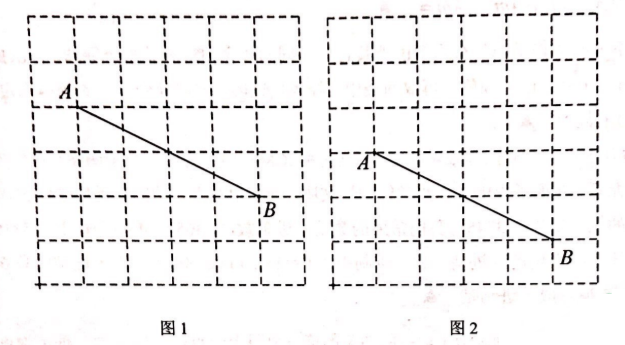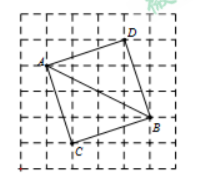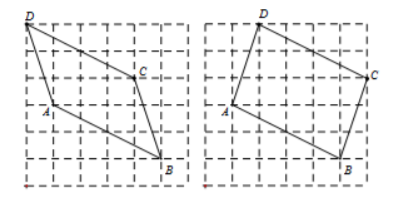(1) 在图 1 的网格内画出以 $A B$ 为对角线的正方形 $A C B D$, 点 $C, D$ 为格点;
(2) 在图 2 的网格内画出以 $A B$ 为边且周长最大的 $\square A B C D$, 点 $C, D$ 为格点(画一个即可).【答案】 (1)(2)下面两图画一个即可。